# The Principles of Electricity

## Current

Current is the flow of electrons. It measures how many electrons per second are moving through a wire. In the circuit we build today, we will have about 20mA of current flowing through the wires. 20mA is equal to 0.020A or 0.020 amps. A 60W light bulb uses about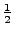A.

Current is measured in units called amps or amperes or A. It is represented by the letter I in equations.

## Voltage

Voltage is a measure of the force that makes electrons want to move from one place to another. It is also known as potential''. If electrons were water droplets, voltage would be the pressure at the base of the water tower. A tall water tower has large pressure at the base; a short tower has little pressure.

At 0V between two points, no electrons will move between those points. No current will flow if those points are connected by a wire. It's like putting a pipe between two ends of a flat lake: no water flows through the pipe.

A small voltage (like 1.5V from an AA battery) is like a fairly flat river. If you put a pipe (wire) between two points along the river, water will flow. But for a given size pipe, the amount of water per second won't be very big.

A high voltage (say, 120V) is like a waterfall along that river. If you put that same pipe between the top and the bottom of that waterfall, water will flow like crazy.

But voltage is not the same as capacity. Say you have a small stream flowing parallel to the river. If you put a small pipe from one point along each waterway to a set distance downstream, you will get the same amount of water flow in each of the two pipes. But if you replace the small water pipe with a big water pipe, the stream won't be able to provide enough water to fill the pipe, and you'll get more water in the river-pipe than the stream-pipe. The stream and the river result in the same drop in water pressure from one point to another (like a voltage), but can provide a different maximum amount of water (just like lower capacity batteries go dead earlier than high capacity batteries).

A battery is rated both with a voltage (like 1.5V) and a capacity (like 1600mAh). A dead battery has run out of charge - it has exhausted its store of electrons. It's like an empty water tower.

Voltage is measured in units called volts or V. It is represented by the letter V in equations.

## Resistance

A resistor resists the flow of current (flow of electrons). It makes it so fewer electrons flow. A big resistor is like using a skinny pipe - you get less water. A small resistor is like using a big wide pipe - water flows easily.

Resistance is measured in units called Ohms or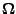. It is represented by the letter R in equations.

## Relationship of V, I, and R

A law called Ohm's Law governs how these quantites relate. It's usually written as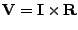. Since we will be interested in calculating the current in our circuit, let's divide both sides by R: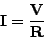Ok, what does this mean? Equations have meanings, you know. Let's say we get high voltage battery. That means V is very big. Let's keep the same size resistor. What happens to the current? If V goes up, the right side of the equation gets bigger, so the left side of the equation must get bigger, so I increases. Making V big is like building a higher water tower. Keeping R the same size is like not changing the size of the water pipes. More water (or electrical current) flows.

# The Electrical Components We Will Use

## Resistors

Resistors resist current flow. In our circuit, they let us regulate how much current flows through the LED. If we want a brightly glowing LED, then we need a lot of current. To allow a lot of current, we don't want to resist the current very much so we pick a resistor with a small resistance.

### The resistor color code

The stripes on a resistor indicate its resistance and tolerance. The resistance indicates how much the resistor will constrict current flow. The tolerance indicates how precisely the resistor's value actually matches what the color code indicates.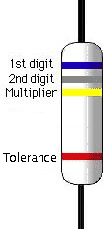The three stripes clumped together indicate the resistance. The first two stripes correspond to the two most significant digits. The third stripe represents the number of zeros. The following table indicates what each color represents:
 black brown red orange yellow green blue violet grey white 0 1 2 3 4 5 6 7 8 9

So for example blue-gray-yellow stripes correspond to 6(blue), 8(gray), and 4 zeros (yellow), which is 680000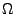: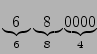. That's a lot of zeros to count, so people usually add prefixes to the units to remove zeros in sets of three. The most common prefixes are k for 3 zeros, and M for 6 zeros. So instead of 680000, we can write 680k.

The fourth stripe that's a little further from the others indicates the tolerance, or how precisely the resistor's value actually matches what the color code indicates. So if you have a 100resistor with a 5% tolerance, that resistor could actually be anywhere between 95to 105. What the resistor companies actually do is they fabricate lots of resistors, then measure them and sort them by tolerance. So in practice, if a resistor has a 5% band on it, its value will be between 95 and 97.99999or between 102.00001 and 105. All the resistors between 98 and 102will have been labelled as 2% resistors by the manufacturer and sold at a higher price. The colors for tolerance are:
 brown red gold silver none 1% 2% 5% 10% 20%

## Batteries

The battery stores energy. It provides a source of electrons. It's like a water tower, in our water analogy. We usually hear batteries described by their voltage - a measure of the strength of the force provided by the battery to make electrons flow from one battery terminal, through a circuit, to the other battery terminal. The batteries we'll be using are 1.5V.

Batteries also differ in how many electrons they store - their capacity - measured in milliamp-hours (mAh). Voltage tells you how strongly the battery pressures the electrons to flow. Capacity tells you how many of those electrons it holds. The capacity of our batteries is about 1650mAh. This means that if we were to use the battery to power one LED, drawing 20mA of current, the battery would last over 80 hours.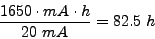We will be using two batteries. There are a couple of ways to connect batteries shown in Fig. 2.2.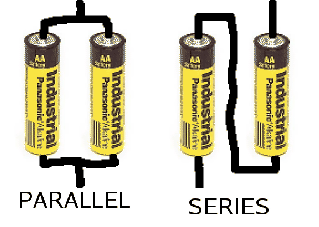Connecting batteries in parallel is like putting two water towers next to each other. The water pressure would be the same as with a single battery (so the voltage doesn't change). But we have twice as much water (so we double the capacity). Connecting batteries in series is like stacking two water towers on top of each other, making a water tower that is twice as tall. We have twice as much water pressure (so the voltage is doubled).

We will connect our batteries in series. The two 1.5V batteries will give us 3V.

## LEDs

LED stands for Light Emitting Diode. A diode is a type of electrical circuit component that lets current flow one way but not the other. It's like a one-way valve. A light-emitting diode puts out light when current flows through it. More current results in more light. Less current gives less light.

The LED component we're using actually contains two LEDs. The red LED faces one way and the green LED faces the other way. So depending on how you connect it in the circuit, one or the other will glow but not both.

# Apply Ohm's Law to our circuit

Ok, so what's going on in our circuit? The batteries provide the source of electrons at a steady voltage of 3V. The LEDs glow brightly if there is a lot of current, not much if there is little current, and not at all if there is no current. The resistor controls how much current flows. Ohm's Law tells us the relationship between these quantities.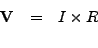Let's do an example calculation.

What do we plug in for V, the voltage? The two batteries are 1.5V each. We've connected them in series. It's like stacking one water tower on top of another: the result is twice as high. So our two 1.5V batteries are like one 3.0V battery. So use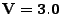.

What do we plug in for R, the resistance? You have three resistors in your kit, each with a different resistance. The colored stripes on the resistor indicate the value of the resistor. One of those resistors is 680. So use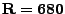. Note that the LED has some resistance of its own. But the resistance of the LED is so low that it gets dwarfed by the resistor, so we can ignore the LEDs resistance.

What do we plug in for I, the current? Well, that's what we calculate! Ohm's Law will tell us how much current (I) to expect given the voltage (V) provided by the battery, and the resistance (R) from the resistor that we choose. It can help us pick the correct resistor. So let's do some math: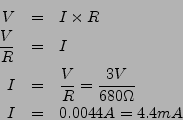That's 4.4mA, which we read out loud as 4.4 milli-amps.

What do you think will happen if we put in a smaller resistor? Look back at: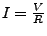. If we make R smaller, that's makes the whole fraction bigger. Look at an example: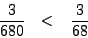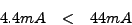So a smaller resistor (R) gives us a bigger current (I). Big current means more light. Try it! Put in a smaller value resistor!

# Let's build our circuit!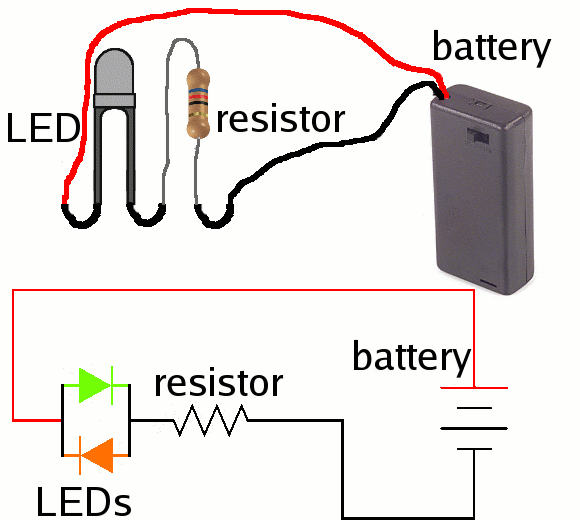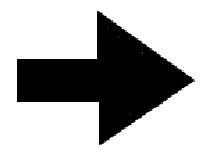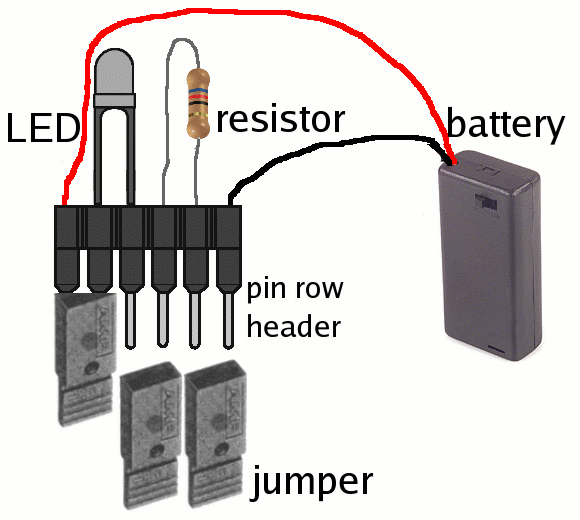ania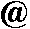alumni.rice.edu
http://ofb.net/~ania
2006-03-08